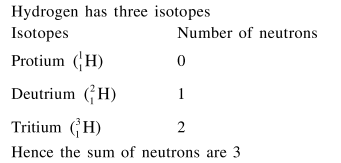# Hydrogen has three isotopes

Question:

Hydrogen has three isotopes $(\mathrm{A}),(\mathrm{B})$ and (C). If the number of neutron(s) in $(A),(B)$ and (C) respectively, are $(x),(y)$ and $(z)$, the sum of $(x)$, (y) an (z) is :

1. 4

2. 3

3. 2

4. 1

Correct Option: , 2

Solution: